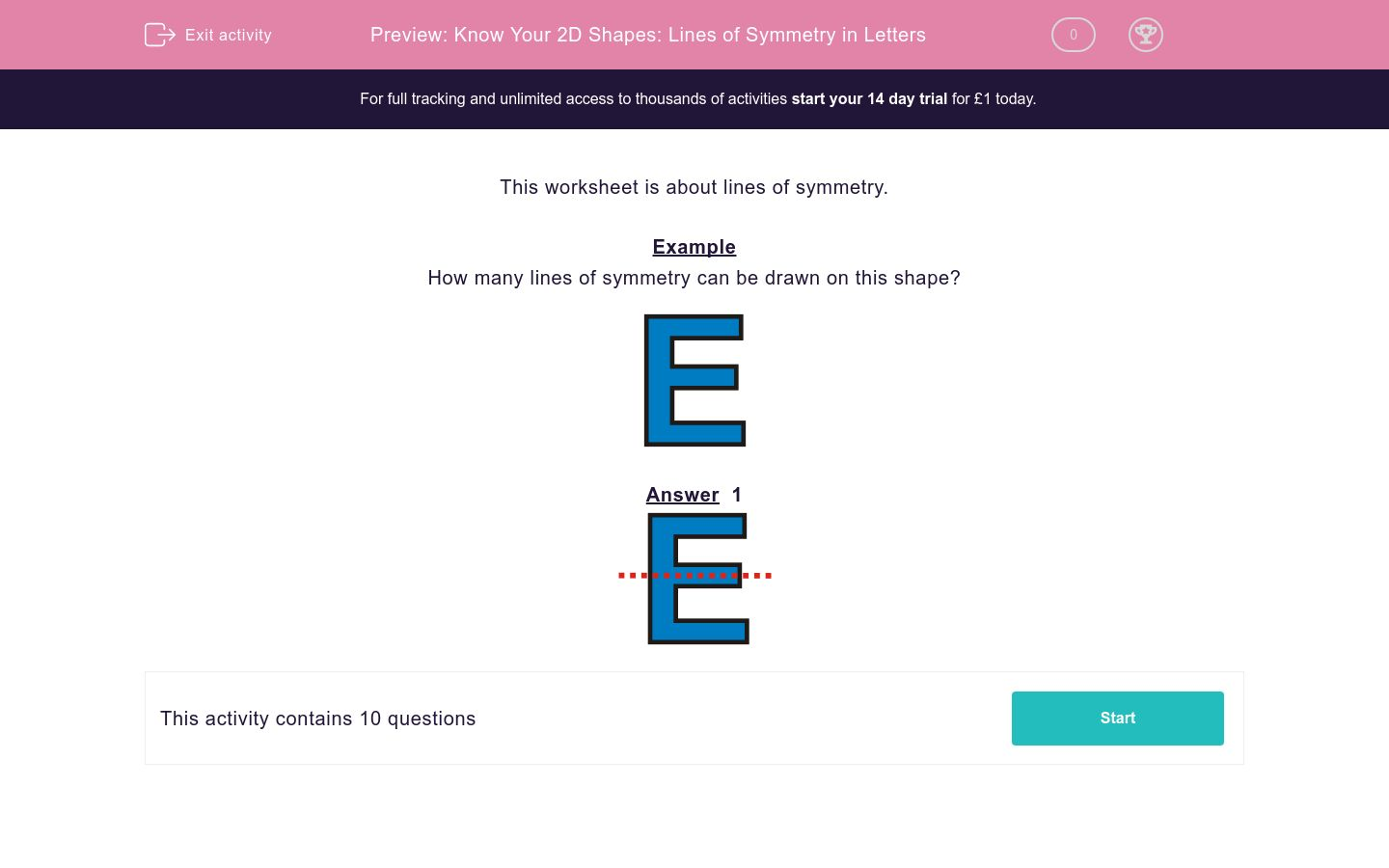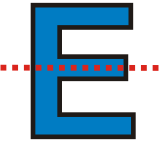# Know Your 2D Shapes: Lines of Symmetry in Letters

In this worksheet, students state how many lines of symmetry a letter has.Key stage:  KS 2

Curriculum topic:   Maths and Numerical Reasoning

Curriculum subtopic:   Symmetry

Difficulty level:### QUESTION 1 of 10

This worksheet is about lines of symmetry.

Example

How many lines of symmetry can be drawn on this shape?How many lines of symmetry can be drawn on this shape?3

2

1

How many lines of symmetry can be drawn on this shape?3

2

1

How many lines of symmetry can be drawn on this shape?3

2

1

How many lines of symmetry can be drawn on this shape?1

2

3

How many lines of symmetry can be drawn on this shape?2

1

3

How many lines of symmetry can be drawn on this shape?3

2

1

How many lines of symmetry can be drawn on this shape?3

2

1

How many lines of symmetry can be drawn on this shape?3

2

1

How many lines of symmetry can be drawn on this shape?3

2

1

How many lines of symmetry can be drawn on this shape?3

2

1

• Question 1

How many lines of symmetry can be drawn on this shape?1
EDDIE SAYS• Question 2

How many lines of symmetry can be drawn on this shape?1
EDDIE SAYS• Question 3

How many lines of symmetry can be drawn on this shape?2
EDDIE SAYS• Question 4

How many lines of symmetry can be drawn on this shape?1
EDDIE SAYS• Question 5

How many lines of symmetry can be drawn on this shape?2
EDDIE SAYS• Question 6

How many lines of symmetry can be drawn on this shape?1
EDDIE SAYS• Question 7

How many lines of symmetry can be drawn on this shape?1
EDDIE SAYS• Question 8

How many lines of symmetry can be drawn on this shape?1
EDDIE SAYS• Question 9

How many lines of symmetry can be drawn on this shape?1
EDDIE SAYS• Question 10

How many lines of symmetry can be drawn on this shape?2
EDDIE SAYS---- OR ----

Sign up for a £1 trial so you can track and measure your child's progress on this activity.

### What is EdPlace?

We're your National Curriculum aligned online education content provider helping each child succeed in English, maths and science from year 1 to GCSE. With an EdPlace account you’ll be able to track and measure progress, helping each child achieve their best. We build confidence and attainment by personalising each child’s learning at a level that suits them.

Get started